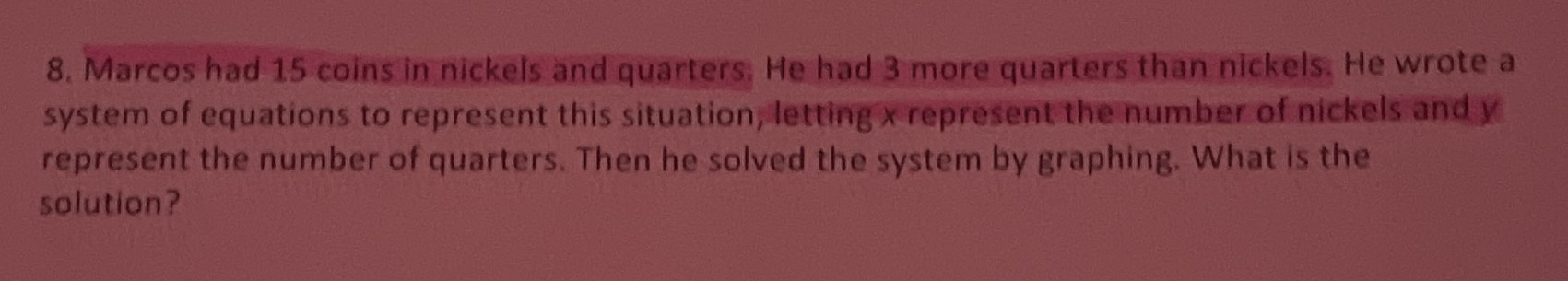### ¿Todavía tienes preguntas de matemáticas?

Pregunte a nuestros tutores expertos
Algebra
Pregunta8. Marcos had $$15$$ coins in nickels and quarlers. He had $$3$$ more quarters than nickels. He wrote a system of equations to represent this situation, letting $$x$$ represent the number of nickels and $$y$$ represent the number of quarters. Then he solved the system by graphing. What is the solution?